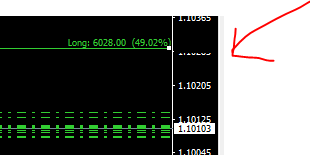# MT4 indicator - lines for profit and loss52

hi, i am trying to add commission and swaps option for my code, i am unable to do so, kindly help.

```#property version       "2.00"

#property description   "The indicator displays profit (loss) on the current symbol."
#property description   "You can freely drag and drop the line to display the current profit or loss."
#property strict
#property indicator_chart_window

enum   choice1 {cash,points};
input  choice1 summ_in=cash;              //To count in money or in pips
enum   choice0 {yes,no};
extern int     MagicNumber=0;             //Magic Number (0 - all orders by symbol)
extern double  offset=200;                //Offset for first print (from average price)
extern color   profit_color=LimeGreen;    //Color profit
extern color   loss_color=DarkOrange;     //Color loss

datetime       time_f1,time_f2;
double         sell_lots,sell_sum_price,sell_sum_comission;
double         summ_stoplimit_price;
string         Label_prefix="lpl_";
int            totalOrders,how_StopLimit;
int            TICKETS_StopLimit[];
//+------------------------------------------------------------------+
//| Custom indicator initialization function                         |
//+------------------------------------------------------------------+
int OnInit()
{
ChartSetInteger(0,CHART_EVENT_MOUSE_MOVE,1);
ChartSetInteger(0,CHART_EVENT_OBJECT_DELETE,1);
offset=NormalizeDouble(offset*_Point,_Digits);
time_f1=Time[Bars-1];
time_f2=Time;

CreateLines();

return(INIT_SUCCEEDED);
}
//+------------------------------------------------------------------+
//| Expert deinitialization function                                 |
//+------------------------------------------------------------------+
void OnDeinit(const int reason)
{
DeleteObjects();
}
//+------------------------------------------------------------------+
//| Custom indicator iteration function                              |
//+------------------------------------------------------------------+
int OnCalculate(const int rates_total,
const int prev_calculated,
const datetime &time[],
const double &open[],
const double &high[],
const double &low[],
const double &close[],
const long &tick_volume[],
const long &volume[],
{
if(how_StopLimit>0)
{
double summ=0;
for(int i=0; i<how_StopLimit; i++)
{
OrderSelectbyTicket(TICKETS_StopLimit[i]);
summ+=OrderOpenPrice();
}
if(summ_stoplimit_price!=summ)
{summ_stoplimit_price=summ; CreateLines();}
}

if(totalOrders!=OrdersTotal()) CreateLines();

return(rates_total);
}
//+------------------------------------------------------------------+
//| ChartEvent function                                              |
//+------------------------------------------------------------------+
void OnChartEvent(const int id,
const long &lparam,
const double &dparam,
const string &sparam)
{

///move long
{
{
}
}

///move short
if(price_sell_line>0 && id==CHARTEVENT_MOUSE_MOVE && sparam=="1" && price_sell_line!=NormalizeDouble(ObjectGetDouble(0,Label_prefix+"lsell",OBJPROP_PRICE,0),_Digits))
{
if(ObjectFind(Label_prefix+"lsell")>=0)
{
price_sell_line=NormalizeDouble(ObjectGetDouble(0,Label_prefix+"lsell",OBJPROP_PRICE,0),_Digits);
SetLevel("sell");
}
}

//check delete
if(id==CHARTEVENT_OBJECT_DELETE)
{
CreateLines();
}

}
//+------------------------------------------------------------------+
//|                                                                  |
//+------------------------------------------------------------------+
void CreateLines()
{
double Tick_Value=NormalizeDouble(MarketInfo(_Symbol,MODE_TICKVALUE),_Digits);

CalcPosition();

{
}

if(sell_lots>0)
{
sell_avg_price=NormalizeDouble(sell_sum_price/sell_lots-(sell_sum_comission/sell_lots/Tick_Value*_Point),_Digits);
if(ObjectFind(Label_prefix+"lsell")>=0) price_sell_line=NormalizeDouble(ObjectGetDouble(0,Label_prefix+"lsell",OBJPROP_PRICE,0),_Digits);
if(price_sell_line<=0) price_sell_line=sell_avg_price-offset;
SetLevel("sell");
}
}
//+------------------------------------------------------------------+
//|                                                                  |
//+------------------------------------------------------------------+
void SetLevel(string prefix)
{
double profit;
double Tick_Value=NormalizeDouble(MarketInfo(_Symbol,MODE_TICKVALUE),_Digits);

if(prefix=="sell") profit=((summ_in==cash)?(sell_avg_price-price_sell_line)/_Point*sell_lots*Tick_Value:(sell_avg_price-price_sell_line)/_Point);

double procent=profit/(AccountBalance()/100);

string new_txt=((prefix=="sell")?"Short: ":"Long: ")+DoubleToString(profit,((summ_in==cash)?2:0))+"  ("+DoubleToString(procent,2)+"%)";
color cvet=((profit>=_Point/2) ? profit_color : loss_color);

}
//+------------------------------------------------------------------+
//|                                                                  |
//+------------------------------------------------------------------+
void SetHLine(
string   lines_name,
double   lines_price
)
{
if(ObjectFind(lines_name)<0)
{
ObjectCreate(0,lines_name,OBJ_HLINE,0,0,lines_price);
ObjectSetInteger(0,lines_name,OBJPROP_COLOR,clrNONE);
ObjectSetInteger(0,lines_name,OBJPROP_WIDTH,1);
ObjectSetInteger(0,lines_name,OBJPROP_SELECTABLE,true);
ObjectSetInteger(0,lines_name,OBJPROP_SELECTED,true);
ObjectSetInteger(0,lines_name,OBJPROP_BACK,false);
ObjectSetInteger(0,lines_name,OBJPROP_ZORDER,10);
ObjectSetInteger(0,lines_name,OBJPROP_HIDDEN,true);
ObjectSetString(0,lines_name,OBJPROP_TOOLTIP,"\n");
}
else
{
ObjectSetDouble(0,lines_name,OBJPROP_PRICE1,lines_price);
}
}
//+------------------------------------------------------------------+
//|                                                                  |
//+------------------------------------------------------------------+
void SetFibo(const string   nname,
double         price1,
color          cvet,
string         text
)
{
if(ObjectFind(nname)<0)
{
ObjectCreate(0,nname,OBJ_FIBO,0,time_f1,price1,time_f2,price1);
ObjectSetInteger(0,nname,OBJPROP_COLOR,clrNONE);
ObjectSetInteger(0,nname,OBJPROP_STYLE,STYLE_SOLID);
ObjectSetInteger(0,nname,OBJPROP_WIDTH,1);
ObjectSetInteger(0,nname,OBJPROP_SELECTABLE,false);
ObjectSetInteger(0,nname,OBJPROP_SELECTED,false);
ObjectSetInteger(0,nname,OBJPROP_BACK,true);
ObjectSetInteger(0,nname,OBJPROP_RAY_RIGHT,true);
ObjectSetInteger(0,nname,OBJPROP_HIDDEN,true);
ObjectSetString(0,nname,OBJPROP_TOOLTIP,"\n");
ObjectSetInteger(0,nname,OBJPROP_LEVELS,1);
ObjectSetInteger(0,nname,OBJPROP_LEVELCOLOR,0,cvet);
ObjectSetInteger(0,nname,OBJPROP_LEVELWIDTH,0,1);
ObjectSetInteger(0,nname,OBJPROP_LEVELSTYLE,0,STYLE_SOLID);
ObjectSetString(0,nname,OBJPROP_LEVELTEXT,0,text);
}
else
{
ObjectSetInteger(0,nname,OBJPROP_LEVELCOLOR,0,cvet);
ObjectSetInteger(0,nname,OBJPROP_TIME1,time_f1);
ObjectSetDouble(0,nname,OBJPROP_PRICE1,price1);
ObjectSetDouble(0,nname,OBJPROP_PRICE2,price1);
ObjectSetString(0,nname,OBJPROP_LEVELTEXT,0,text);
}

}
//+------------------------------------------------------------------+
//|                                                                  |
//+------------------------------------------------------------------+
void DeleteObjects()
{
for(int i=ObjectsTotal()-1;i>=0;i--)
{
string name=ObjectName(i);
if(StringFind(name,Label_prefix,0)>=0)
ObjectDelete(0,name);
}
}
//+------------------------------------------------------------------+
//|                                                                  |
//+------------------------------------------------------------------+
void CalcPosition()
{
totalOrders=OrdersTotal();
sell_lots=sell_sum_price=sell_sum_comission=0;
how_StopLimit=0;
ArrayResize(TICKETS_StopLimit,0);

if(totalOrders>0)
{
for(int i=0;i<totalOrders;i++)
{
if(OrderSymbol()==_Symbol && (MagicNumber==0 || MagicNumber==OrderMagicNumber()) && (add_pending==yes || OrderType()<2))
{
{

{
how_StopLimit++;
ArrayResize(TICKETS_StopLimit,how_StopLimit);
TICKETS_StopLimit[how_StopLimit-1]=OrderTicket();
}

}
if(OrderType()==OP_SELL || OrderType()==OP_SELLLIMIT || OrderType()==OP_SELLSTOP)
{
sell_lots+=OrderLots();
sell_sum_price+=OrderOpenPrice()*OrderLots();
sell_sum_comission+=OrderSwap()+OrderCommission();

if(OrderType()==OP_SELLLIMIT || OrderType()==OP_SELLSTOP)
{
how_StopLimit++;
ArrayResize(TICKETS_StopLimit,how_StopLimit);
TICKETS_StopLimit[how_StopLimit-1]=OrderTicket();
}
}
}
}
}
if(sell_lots==0) {ObjectDelete(0,Label_prefix+"lsell"); ObjectDelete(0,Label_prefix+"fsell"); price_sell_line=0;}
}
//+------------------------------------------------------------------+
//|                                                                  |
//+------------------------------------------------------------------+
void OrderSelectbyTicket(int ord_ticket)
{
bool i2=false;
while(i2==false && !IsStopped())
{
i2=OrderSelect(ord_ticket,SELECT_BY_TICKET);
}
}
//+------------------------------------------------------------------+
```52

with few adjustment that i need, i also observed one error, which in code it is clear but appearing on display, the price shown in image is getting overlap by a black block, kindly help me to resolve it please.52

please i need help over this error, kindly help, price is not showing.